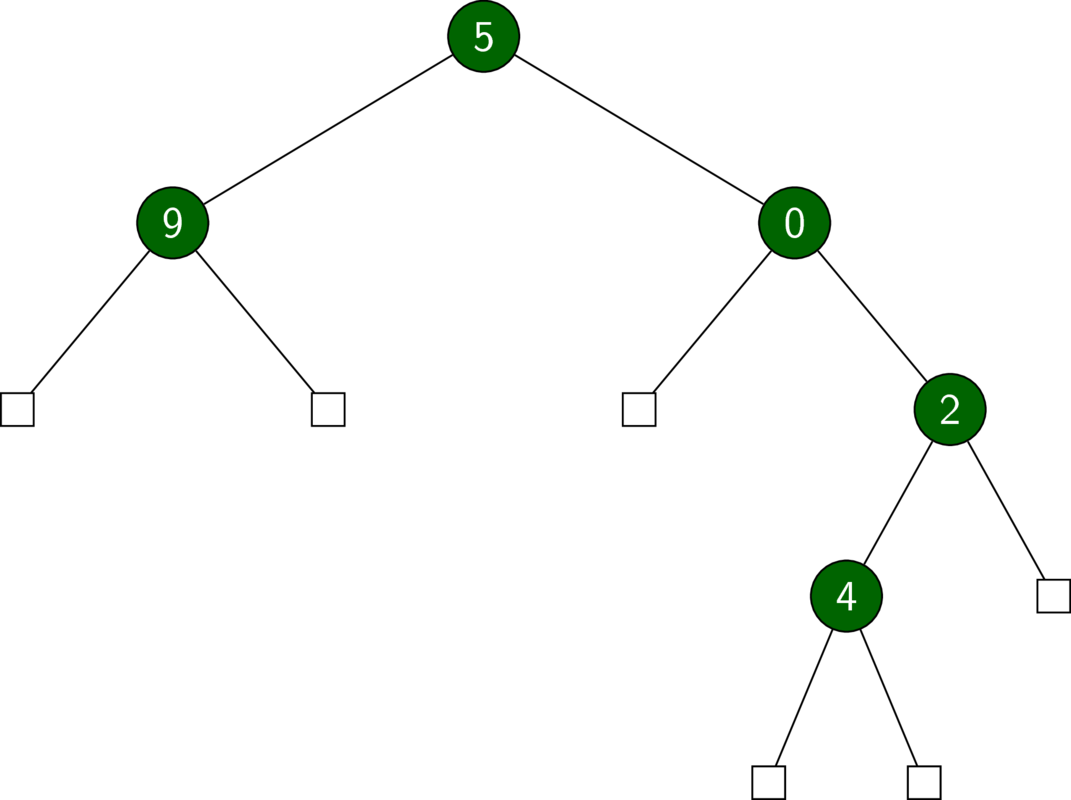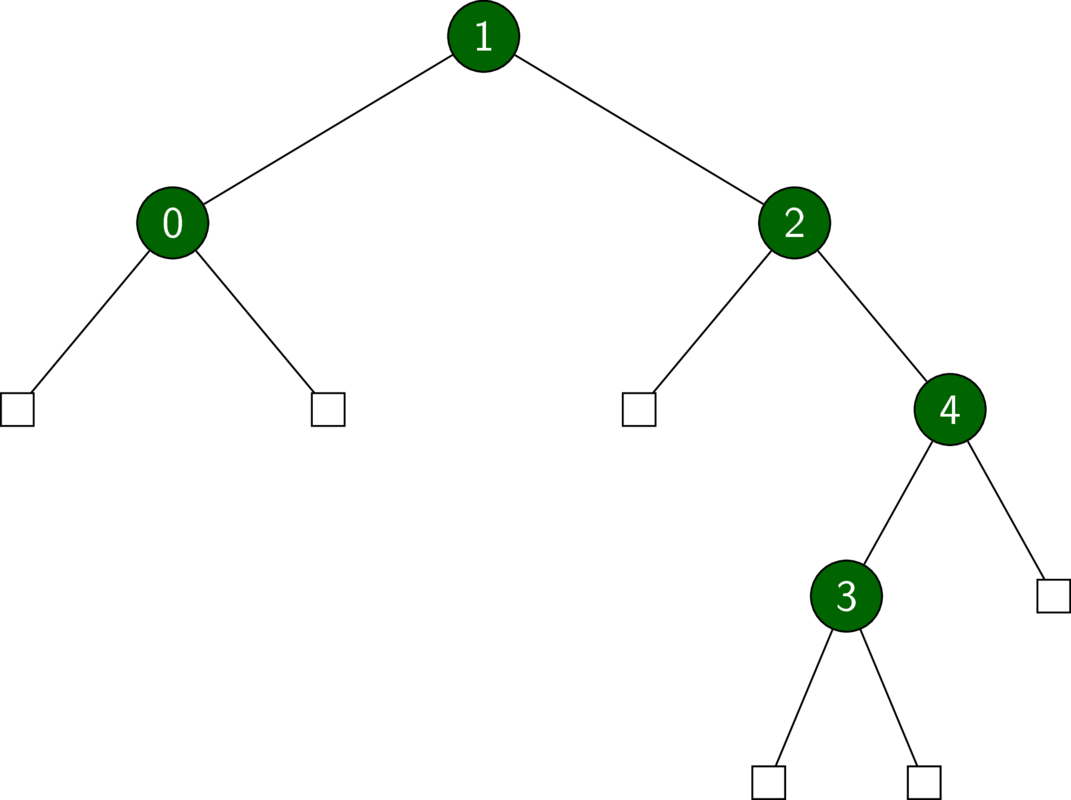# Okasaki's PFDS, Chapter 2

This post contains my solutions to the exercises in chapter 2 of Okasaki’s ‘Purely Functional Data Structures’. The latest source code can be found in my GitHub repo.

## Exercise 2.1

Write a function suffixes of type

suffixes :: [a] -> [[a]]

that takes a list xs and returns a list of all the suffixes of xs in decreasing order of length. For example,

suffixes [1, 2, 3, 4] == [[1, 2, 3, 4], [2, 3, 4], [3, 4], , []]

Show that the resulting list of suffixes can be generated in $$\mathcal O (n)$$ time and represented in $$\mathcal O (n)$$ space.

## Solution 2.1

Since there are as many recursive calls to suffixes as there are elements of xs, and both : and tail run in constant time, suffixes must be linear in the length of the list xs. That is, the solution to the recursion $$T (n) = T(n-1) + \mathcal O (1)$$ is $$T (n) = \mathcal O (n)$$.

Moreover, we use the $$n$$ lists that already exist and also $$\mathcal O (n)$$ new pointers to each of those lists. Therefore, suffixes xs is represented in $$\mathcal O (n)$$ space.

## Binary Search Trees (BSTs)

We start with binary trees (not search trees yet!). A binary tree is either empty or has two children, which we call ‘left’ and ‘right’.

data Tree a = E
| T (Tree a) a (Tree a)
deriving (Show, Eq)

In the binary trees below, empty nodes are depicted as squares.A binary tree (which is NOT a BST).

A BST is a binary tree with some extra structure. In particular, we require that the key of a node be greater than that of any of its left descendants and smaller than that of any of its right descendants.A binary tree which IS a BST.

In the following exercises, we implement BSTs in the context of sets.

## Exercise 2.2

Rewrite member to take no more than $$d+1$$ comparisons.

See source.

## Exercise 2.3

Rewrite insert using exceptions to avoid copying the entire search path (in the case of inserting an existing element).

## Solution 2.3

See source.

We use the Maybe data structure for our errors, i.e. Nothing indicates an error.

## Exercise 2.4

Combine the ideas of Exercise 2.2 and Exercise 2.3 to create an insert function that performs no unnecessary copying and uses no more than $$d+1$$ comparisons.

See source.

## Exercise 2.5

1. Write a function complete of type

complete :: a -> Int -> UnbalancedSet a

such that complete a d is a complete binary tree of depth d with the key a at every node. This function should run in $$\mathcal O (d)$$ time.

2. Extend complete to create balanced trees of arbitrary size that runs in $$\mathcal O (n)$$ time, where $$n$$ is the number of nodes. A tree is said to be balanced if the size of any node’s left child differs by at most one from the size of that node’s right child.

## Solution 2.5

See source.

Item 1.

The recursion is given by $$T(d) = T(d-1) + \mathcal O (1)$$, which is solved by $$T (d) = \mathcal O (d)$$.

Item 2.

The complexity of balance is equal to that of create2. The recursion for create2 is given by $$T (n) = T (n/2) + \mathcal O (1)$$, which is solved by $$T (n) = \mathcal O (\log n)$$.

## Exercise 2.6

Adapt the UnbalancedSet functor to support finite maps rather than sets. The signature for finite maps is as follows.

class FiniteMap m k a where
empty  :: m k a
bind   :: k ->     a -> m k a -> m k a
lookup :: k -> m k a -> Maybe a

## Solution 2.6

See source.

We reuse the set implementation for UnbalancedSet by creating the Binding data type with the appropriate ordering. Note that this doesn’t allow updating key-value pairs.